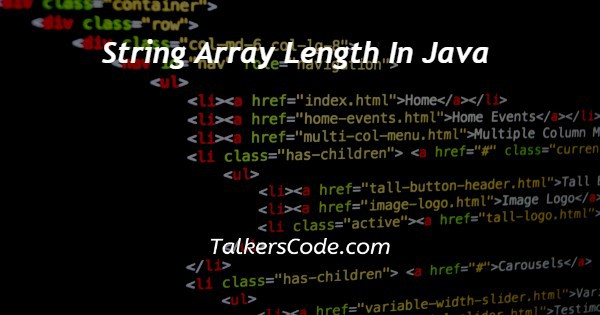# String Array Length In Java

Last Updated : Jul 1, 2023In this article we will show you the solution of string array length in java, a java array's length attribute indicates how many elements it contains. The length of an array cannot be found by any predefined method in java.

The length attribute, on the other hand, is useful if you're trying to find out how long a given array is. a collection's length, or size, indicates how many elements are contained within it, or the total number of elements.

It is also possible to learn more about arrays in java by taking a free online course on java programming.

An array's length function, whether it contains strings or numbers, counts the total number of elements. In the length attribute, the array's length is specified.

The array length attribute can be used in many different contexts, including:

• The array must have a minimum value.
• Finding the maximum number within an array.
• On any specific element of the array, you can retrieve its value.
• Checks if there is a particular value in the array.
• In java, there are other possible scenarios where array length can be used.

In the array, there is a function called 'findvalue' that finds the specific value we're searching for.

For each element in the array, we traversed the array in a for loop to determine if it contained the value.

For either case, we gave two statements, such as if it contained the value or not.

In the case of finding the value in the array, It returns true, In the case of not finding it, It returns false. consequently, It returned true in our case or with the statements we provided because we had c++ and python in our array.

A dot and the keyword length() are used to determine the length of a string enclosed in double quotes.

This length() function produces a string as an output, So in java you need to store it as an integer.

The length of the string is then stored in an integer array named length array. Array.length() is used to set this length array variable.

## Step By Step Guide On String Array Length In Java :-

```import java.util.*;
class main
{
public static void main(string[] args)
{
integer[] intarray = {1,5,7,9};
string[] strarray = { "one", "two", "three", “four” };
system.out.println("functions of integer array : " + arrays.tostring(intarray));
system.out.println("the length of the array is : " + intarray.length);
system.out.println("functions of string array : " + arrays.tostring(strarray));
system.out.println("the length of the string array is : " + strarray.length);
}
}```
1. The first step is to start a program with an import.
2. Next, a program execution class needs to be created.
3. Our next step is to define the public static void main function.
4. Arrays containing integers and strings are defined in the next step.
5. The program is then closed using the system.out.printin method.

## Conclusion :-

Array elements are counted using the length attribute in java.

An array's length cannot be determined using a predefined method in java. Arrays can be measured by using their length attribute.

We can call the length of an array or its size the total number of elements in it when we declare an array.

I hope this article on string array length in java helps you and the steps and method mentioned above are easy to follow and implement.